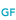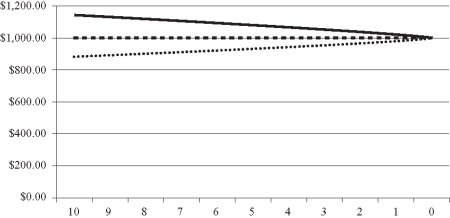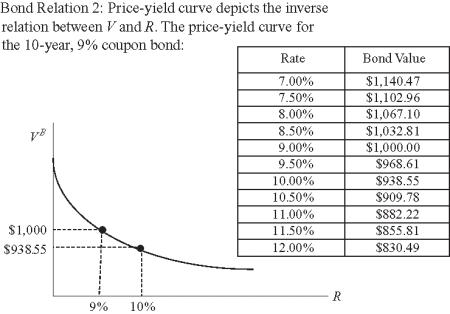# Bond price relations

Learn how a bond's pricing can be influenced by interest rate changes, coupon rates, values, and more.

•WILEY GLOBAL FINANCE
• Bonds
• Bonds
• Bonds
• Bonds

## Relation between coupon rate, required rate, value, and par value

The value of a bond—in our example here, a corporate bond with a face value of \$1,000 and a coupon equal to 9% of par each year, for an investor who requires a 10% annual return—is not equal to its par value. This can be explained by the fact that the discount rate and coupon rate are different.

• Specifically, for investors in the above case to obtain the 10% rate per year from a bond promising to pay an annual rate of CR = 9% of par, they would have to buy the bond at a value, or price, below par: The bond would have to be purchased at a discount from its par, Vob < F.
• In contrast, if the coupon rate is equal to the discount rate (i.e., R = 9%), then the bond's value would be equal to its par value, Vob = F. In this case, investors would be willing to pay \$1,000 for this bond, with each investor receiving \$90 each year in coupons.
• Finally, if the required rate is lower than the coupon rate, then investors would be willing to pay a premium over par for the bond, Vob > F. This might occur if bonds with comparable features were trading at rates below 9%. In this case, investors would be willing to pay a price above \$1,000 for a bond with a coupon rate of 9%.

Thus, the first relationship to note is that a bond's value (or price) will be equal to, greater than, or less than its face value depending on whether the coupon rate is equal to, less than, or greater than the required rate.

Bond-Price Relation 1

If CR = R => Vb = F: Bond valued at par

If CR < R => Vb < F: Bond valued at par

If CR > R => Vb > F: Bond valued at par

In addition to the preceding relations, the relation between the coupon rate and required rate also explains how the bond's value changes over time.

• If the required rate is constant over time, and if the coupon rate is equal to it (i.e., the bond is priced at par), then the value of the bond will always be equal to its face value throughout the life of the bond. This is illustrated in the graph below by the horizontal line that shows the value of the 9% coupon bond is always equal to the par value. Here investors would pay \$1,000 regardless of the terms to maturity.
• On the other hand, if the required rate is constant over time and the coupon rate is less (i.e., the bond is priced at a discount), then the value of the bond will increase as it approaches maturity; if the required rate is constant and the coupon rate is greater (i.e., the bond is priced at a premium), then the value of the bond will decrease as it approaches maturity. These relationships are also illustrated in the graph below.

### The value over time of an original 10-year, 9% annual coupon bond selling at par, discount, and premium## Relation between value and rate of return

Given known coupon and principal payments, the only way an investor can obtain a higher rate of return on a bond is for its price (value) to be lower. In contrast, the only way for a bond to yield a lower rate is for its price to be higher. Thus, the second bond relationship to note is that there is an inverse relationship between the price and rate of return on a bond.

Bond-Price Relation 2

If R Increases => VoDecreases

If R Decreases => Vob  Increases

The inverse relation between a bond's price and rate of return is illustrated by the negatively sloped price-yield curve shown in the graph below. The curve shows the different values of a 10-year, 9% annual coupon bond given different rates. As shown, the 10-year bond has a value of \$938.55 when R = 10% and \$1,000 when R = 9%. In addition to showing a negative relation between price and yield, the price-yield curve is also convex from below (bow shaped). This convexity implies that for equal increases in yields, the value of the bond decreases at a decreasing rate (for equal decreases in yields, the bond's price increases at an increasing rate). The bow shape of a bond's price-yield curve is referred to as the bond's convexity. It has important implications related to bond investment and management.

### Price-Yield relation, 10-year, 9% annual coupon bond## The relation between a bond's price sensitivity to interest rate changes and term to maturity

The third bond relationship to note is the relation between a bond's price sensitivity to interest rate changes and its maturity. Specifically:

Bond-Price Relation 3

The greater the bond's term to maturity, the greater its price sensitivity to a given change in interest rates.

This relationship can be seen by comparing the price sensitivity to interest rate changes of the 10-year, 9% coupon bond in our above example with a 1-year, 9% coupon bond. As shown in the chart below, if the required rate is 10%, then the 10-year bond would trade at \$938.55, whereas the 1-year bond would trade at \$990.91 (\$1,090/1.10). If the interest rate decreases to 9% for each bond (a 10% change in rates), both bonds would increase in price to \$1,000. For the 10-year bond, the percentage increase in price would be 6.55% [(\$1,000 − \$938.55)/\$938.55], whereas the percentage increase for the 1-year bond would be only 0.9%. Thus, the 10-year bond's price is more sensitive to the interest rate change than the 1-year bond. In addition, the greater price sensitivity of longer maturity bonds also implies that their price-yield curves are more convex than the price-yield curves for smaller maturity bonds.

## The relation between a bond's price sensitivity to interest rate changes and coupon payments

Consider two 10-year bonds, each priced at a discount rate of 10% and each paying a principal of \$1,000 at maturity, but with one bond having a coupon rate of 10% and priced at \$1,000, and the other having a coupon rate of 2% and priced at \$508.43:

Now suppose that the rate required on each bond decreases to a new level of 9%. The price on the 10% coupon bond, in turn, would increase by 6.4% to equal \$1,064.18, whereas the price on the 2% coupon bond would increase by 8.3% to \$550.76:

In this case, the lower coupon bond's price is more responsive to a given interest rate change than the price of the higher coupon bond. Thus:

Bond-Price Relation 4

The lower a bond's coupon rate, the greater its price sensitivity to changes in discount rates.